• Posts tagged "S4"

# Blog Archives

## R语言游戏框架设计

R的极客理想系列文章，涵盖了R的思想，使用，工具，创新等的一系列要点，以我个人的学习和体验去诠释R的强大。

R语言作为统计学一门语言，一直在小众领域闪耀着光芒。直到大数据的爆发，R语言变成了一门炙手可热的数据分析的利器。随着越来越多的工程背景的人的加入，R语言的社区在迅速扩大成长。现在已不仅仅是统计领域，教育，银行，电商，互联网….都在使用R语言。

• 张丹(Conan), 程序员Java,R,PHP,Javascript
• weibo：@Conan_Z
• blog: http://blog.fens.me
• email: bsspirit@gmail.com1. 贪食蛇的面向对象改造
2. 游戏框架定义
3. 在框架中重新实现贪食蛇游戏

## 1. 贪食蛇的面向对象改造

1.1 定义Snake类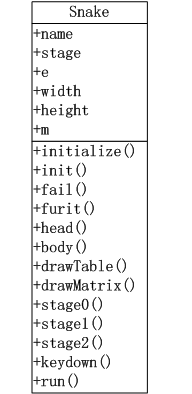• name:游戏的名字
• stage:当前的游戏场景
• e:游戏中的变量，environment类型
• m:游戏地图矩阵
• width:矩阵的宽
• height:矩阵的高

• initialize():构建函数，用于RC类的初始化
• init():给stage1场景初始化游戏变量
• fail():失败查询
• furit():判断并生成水果坐标
• body():生成蛇尾移动坐标。
• drawTable():绘制游戏背景。
• drawMatrix():绘制游戏矩阵。
• stage0():创建开机场景，可视化输出。
• stage1():创建游戏场景，stage1()函数内部，封装了游戏场景运行时的函数，并进行调用。
• stage2():创建结束场景，可视化输出
• keydown():监听键盘事件。
• run():启动函数

1.2 全局函数调用顺序图

1. 通过run()函数启动游戏，进入stage0场景，注册键盘事件。
2. 在stage0场景按任意键切换到stage1场景。
3. init()出始化stage1场景的游戏变量。
4. stage1()运行游戏
5. 当游戏失败fail()或按q键
6. 游戏进行stage2场景，显示游戏结束画面，
7. 按空格键回到stage0重新开始，按q键退出程序。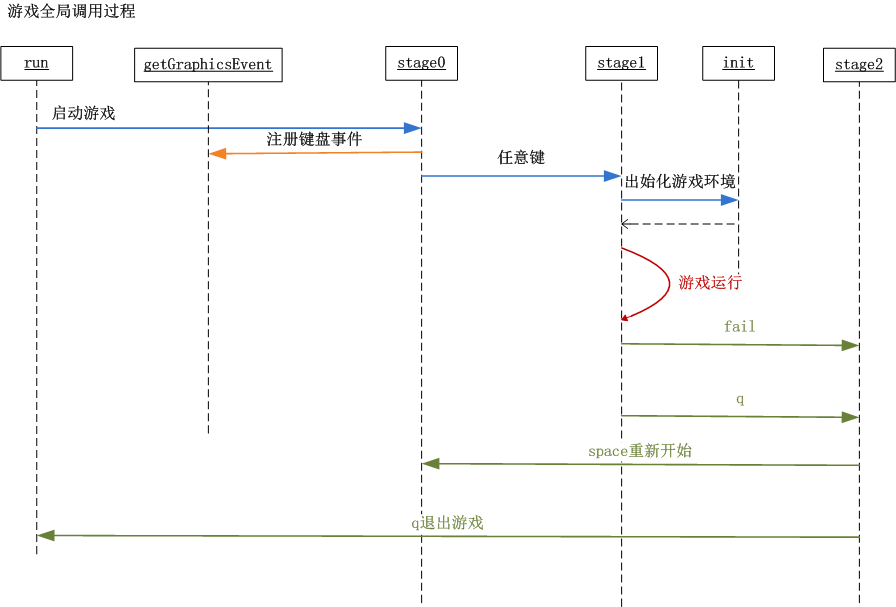1.3 stage1场景游戏环境函数调用顺序图

stage1场景游戏环境函数调用关系。

1. 游戏进入stage1场景，按上下左右(up,down,left,right)方向键操作蛇头的前进路线。
2. furit()函数检查，如果地图上水果被吃掉，生成一个新水果，记录到矩阵中。
4. fail()函数失败检查，no未失败继续，yes失败进行stage2场景。
5. body()函数，蛇身体移动，记录到矩阵中。
6. drawTable()函数，画出游戏背景画布。
7. drawMatrix()函数，画出游戏矩阵。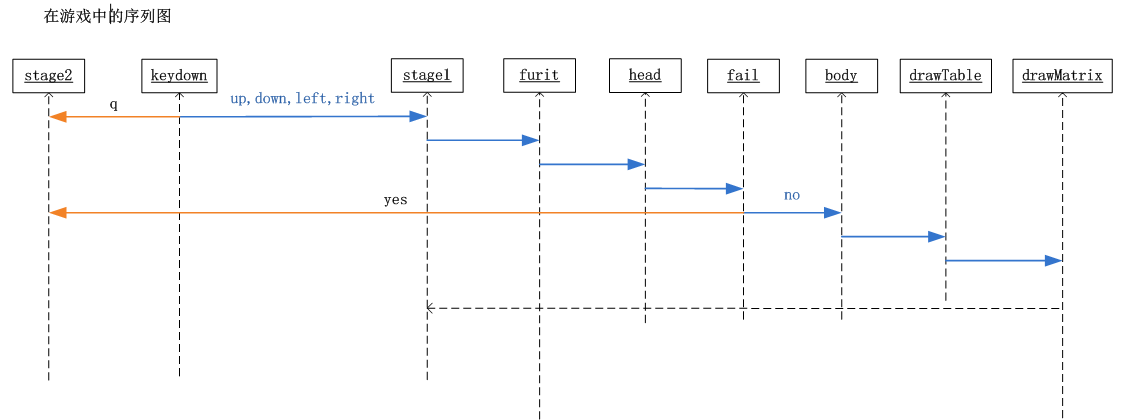## 2. 游戏框架定义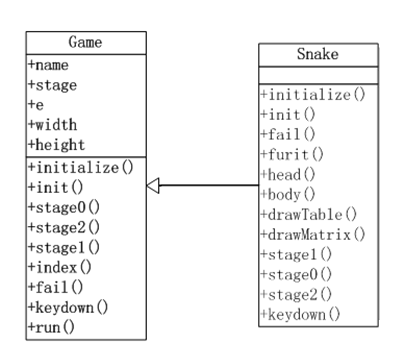• Game类公共属性，包括了所有的Snake类的属性，这是因为这些属性都是全局的，其他的游戏也会用到，而且每个游戏中的属性，可以在e中进行定义。
• Game类公共方法，包括了游戏全局调用的方法，但不包括Snake游戏stage1场景中运行的方法。在Game类的方法中，我们主要实现的都是开发的辅助功能。
• Snake类方法，同样还有Game类的方法，这是用到方法的重写技术。子类的方法，先调用父类的同名方法，然后再执行子类方法里的程序。

## 3. 在框架中重新实现贪食蛇游戏

Game类的代码实现，用R语言的RC面向对象编程进行代码编写。

``````
Game<-setRefClass('Game',

fields=list(
# 系统变量
name="character", # 名字
debug='logical',  # 调试状态
width='numeric',  # 矩阵宽
height='numeric', # 矩阵高

# 应用变量
stage='numeric',  # 场景
e='environment',  # 环境空间变量
m='matrix',       # 数据矩阵
isFail='logical'  # 游戏失败
),

methods=list(

# 构造函数
initialize = function(name,width,height,debug) {
name<<-"R Game Framework"
debug<<-FALSE
width<<-height<<-20   #矩阵宽高
},

# 初始化变量
init = function(){
e<<-new.env()   #环境空间
m<<-matrix(rep(0,width*height),nrow=width)  #数据矩阵
isFail<<-FALSE
},

# 开机画图
stage0=function(){
stage<<-0
init()
},

# 结束画图
stage2=function(){
stage<<-2
},

# 游戏中
stage1=function(default=FALSE){
stage<<-1
if(FALSE){  # 默认游戏中界面
plot(0,0,xlim=c(0,1),ylim=c(0,1),type='n',xaxs="i", yaxs="i")
text(0.5,0.7,label="Playing",cex=5)
}
},

# 矩阵工具
index = function(col) {
return(which(m==col))
},

# 失败操作
fail=function(msg){
print(paste("Game Over",msg))
isFail<<-TRUE
keydown('q')
return(NULL)
},

# 键盘事件，控制场景切换
keydown=function(K){
if(stage==0){ #开机画面
stage1()
return(NULL)
}

if(stage==2){ #结束画面
if(K=="q") q()
else if(K==' ') stage0()
return(NULL)
}
},

# 启动程序
run=function(){
par(mai=rep(0,4),oma=rep(0,4))
stage0()
getGraphicsEvent(prompt="Snake Game",onKeybd=function(K){
if(debug) print(paste("keydown",K))
return(keydown(K))
})
}
)
)
``````

Snake类的代码实现，继承Game类，并实现贪食蛇游戏的私有方法。

``````
# 引用game.r文件
source(file="game.r")

# Snake类，继承Game类
Snake<-setRefClass("Snake",contains="Game",

methods=list(

# 构造函数
initialize = function(name,width,height,debug) {
callSuper(name,width,height,debug) # 调父类

name<<-"Snake Game"
},

# 初始化变量
init = function(){
callSuper()  # 调父类

e\$step<<-1/width #步长
e\$dir<<-e\$lastd<<-'up' # 移动方向
e\$lastx<<-e\$lasty<<-2 # 蛇头上一个点坐标
e\$tail<<-data.frame(x=c(),y=c())#初始蛇尾坐标

e\$col_furit<<-2 #水果颜色
e\$col_tail<<-8 #蛇尾颜色
e\$col_path<<-0 #路颜色
e\$col_barrier<<-1 #障碍颜色
},

# 失败检查
lose=function(){
fail("Out of ledge.")
return(NULL)
}

return(NULL)
}
},

# 随机的水果点
furit=function(){
if(length(index(e\$col_furit))<=0){ #不存在水果
idx<-sample(index(e\$col_path),1)

fx<-ifelse(idx%%width==0,10,idx%%width)
fy<-ceiling(idx/height)
m[fx,fy]<<-e\$col_furit

if(debug){
print(paste("furit idx",idx))
print(paste("furit axis:",fx,fy))
}
}
},

# 方向操作
},

# snake body
body=function(){
if(isFail) return(NULL)

m[e\$lastx,e\$lasty]<<-e\$col_path
if(length(index(e\$col_furit))<=0){ #不存在水果
e\$tail<<-rbind(e\$tail,data.frame(x=e\$lastx,y=e\$lasty))
}

if(nrow(e\$tail)>0) { #如果有尾巴
e\$tail<<-rbind(e\$tail,data.frame(x=e\$lastx,y=e\$lasty))
m[e\$tail[1,]\$x,e\$tail[1,]\$y]<<-e\$col_path
e\$tail<<-e\$tail[-1,]
m[e\$lastx,e\$lasty]<<-e\$col_tail
}

if(debug){
}
},

# 画布背景
drawTable=function(){
if(isFail) return(NULL)

plot(0,0,xlim=c(0,1),ylim=c(0,1),type='n',xaxs="i", yaxs="i")

if(debug){
# 显示背景表格
abline(h=seq(0,1,e\$step),col="gray60") # 水平线
abline(v=seq(0,1,e\$step),col="gray60") # 垂直线
# 显示矩阵
df<-data.frame(x=rep(seq(0,0.95,e\$step),width),y=rep(seq(0,0.95,e\$step),each=height),lab=seq(1,width*height))
text(df\$x+e\$step/2,df\$y+e\$step/2,label=df\$lab)
}
},

# 根据矩阵画数据
drawMatrix=function(){
if(isFail) return(NULL)

idx<-which(m>0)
px<- (ifelse(idx%%width==0,width,idx%%width)-1)/width+e\$step/2
py<- (ceiling(idx/height)-1)/height+e\$step/2
pxy<-data.frame(x=px,y=py,col=m[idx])
points(pxy\$x,pxy\$y,col=pxy\$col,pch=15,cex=4.4)
},

# 游戏场景
stage1=function(){
callSuper()

furit()
lose()
body()
drawTable()
drawMatrix()
},

# 开机画图
stage0=function(){
callSuper()
plot(0,0,xlim=c(0,1),ylim=c(0,1),type='n',xaxs="i", yaxs="i")
text(0.5,0.7,label=name,cex=5)
text(0.5,0.4,label="Any keyboard to start",cex=2,col=4)
text(0.5,0.3,label="Up,Down,Left,Rigth to control direction",cex=2,col=2)
text(0.2,0.05,label="Author:DanZhang",cex=1)
text(0.5,0.05,label="http://blog.fens.me",cex=1)
},

# 结束画图
stage2=function(){
callSuper()
info<-paste("Congratulations! You have eat",nrow(e\$tail),"fruits!")
print(info)

plot(0,0,xlim=c(0,1),ylim=c(0,1),type='n',xaxs="i", yaxs="i")
text(0.5,0.7,label="Game Over",cex=5)
text(0.5,0.4,label="Space to restart, q to quit.",cex=2,col=4)
text(0.5,0.3,label=info,cex=2,col=2)
text(0.2,0.05,label="Author:DanZhang",cex=1)
text(0.5,0.05,label="http://blog.fens.me",cex=1)
},

# 键盘事件，控制场景切换
keydown=function(K){
callSuper(K)

if(stage==1){ #游戏中
if(K == "q") stage2()
else {
if(tolower(K) %in% c("up","down","left","right")){
e\$lastd<<-e\$dir
e\$dir<<-tolower(K)
stage1()
}
}
return(NULL)
}
return(NULL)
}
)
)

snake<-function(){
game<-Snake\$new()
game\$initFields(debug=TRUE)
game\$run()
}

snake()
``````## R语言基于S4的面向对象编程

R的极客理想系列文章，涵盖了R的思想，使用，工具，创新等的一系列要点，以我个人的学习和体验去诠释R的强大。

R语言作为统计学一门语言，一直在小众领域闪耀着光芒。直到大数据的爆发，R语言变成了一门炙手可热的数据分析的利器。随着越来越多的工程背景的人的加入，R语言的社区在迅速扩大成长。现在已不仅仅是统计领域，教育，银行，电商，互联网….都在使用R语言。

• 张丹(Conan), 程序员Java,R,PHP,Javascript
• weibo：@Conan_Z
• blog: http://blog.fens.me
• email: bsspirit@gmail.com

http://blog.fens.me/r-class-s4/S4对象系统具有明显的结构化特征，更适合面向对象的程序设计。Bioconductor社区，以S4对象系统做为基础架构，只接受符合S4定义的R包。

1. S4对象介绍
2. 创建S4对象
3. 访问对象的属性
4. S4的泛型函数
5. 查看S4对象的函数
6. S4对象的使用

## 1 S4对象介绍

S4对象系统是一种标准的R语言面向对象实现方式，S4对象有明确的类定义，参数定义，参数检查，继承关系，实例化等的面向对象系统的特征。

## 2 创建S4对象

• Linux: Ubuntu Server 12.04.2 LTS 64bit
• R: 3.0.1 x86_64-pc-linux-gnu

``````
# 加载pryr包
> library(pryr)
``````

2.1 如何创建S4对象？

2.1.1 setClass()

``````
setClass(Class, representation, prototype, contains=character(),
validity, access, where, version, sealed, package,
S3methods = FALSE, slots)
``````

• Class: 定义类名
• slots: 定义属性和属性类型
• prototype: 定义属性的默认值
• contains=character(): 定义父类，继承关系
• validity: 定义属性的类型检查
• where: 定义存储空间
• sealed: 如果设置TRUE，则同名类不能被再次定义
• package: 定义所属的包
• S3methods: R3.0.0以后不建议使用
• representation R3.0.0以后不建议使用
• access R3.0.0以后不建议使用
• version R3.0.0以后不建议使用

2.2 创建一个S4对象实例

``````
# 定义一个S4对象
> setClass("Person",slots=list(name="character",age="numeric"))

# 实例化一个Person对象
> father<-new("Person",name="F",age=44)

# 查看father对象，有两个属性name和age
> father
An object of class "Person"
Slot "name":
 "F"

Slot "age":
 44

# 查看father对象类型，为Person
> class(father)
 "Person"
attr(,"package")
 ".GlobalEnv"

# 查看father对象为S4的对象
> otype(father)
 "S4"
``````

2.3 创建一个有继承关系的S4对象

``````
# 创建一个S4对象Person
> setClass("Person",slots=list(name="character",age="numeric"))

# 创建Person的子类
> setClass("Son",slots=list(father="Person",mother="Person"),contains="Person")

# 实例化Person对象
> father<-new("Person",name="F",age=44)
> mother<-new("Person",name="M",age=39)

# 实例化一个Son对象
> son<-new("Son",name="S",age=16,father=father,mother=mother)

# 查看son对象的name属性
> son@name
 "S"

# 查看son对象的age属性
> son@age
 16

# 查看son对象的father属性
> son@father
An object of class "Person"
Slot "name":
 "F"

Slot "age":
 44

# 查看son对象的mother属性
> slot(son,"mother")
An object of class "Person"
Slot "name":
 "M"

Slot "age":
 39

# 检查son类型
> otype(son)
 "S4"

# 检查son@name属性类型
> otype(son@name)
 "primitive"

# 检查son@mother属性类型
> otype(son@mother)
 "S4"

# 用isS4()，检查S4对象的类型
> isS4(son)
 TRUE
> isS4(son@name)
 FALSE
> isS4(son@mother)
 TRUE
``````

2.4 S4对象的默认值

``````
> setClass("Person",slots=list(name="character",age="numeric"))

# 属性age为空
> a<-new("Person",name="a")
> a
An object of class "Person"
Slot "name":
 "a"

Slot "age":
numeric(0)

# 设置属性age的默认值20
> setClass("Person",slots=list(name="character",age="numeric"),prototype = list(age = 20))

# 属性age为空
> b<-new("Person",name="b")

# 属性age的默认值是20
> b
An object of class "Person"
Slot "name":
 "b"

Slot "age":
 20
``````

2.5 S4对象的类型检查

``````
> setClass("Person",slots=list(name="character",age="numeric"))

# 传入错误的age类型
Error in validObject(.Object) :
invalid class “Person” object: invalid object for slot "age" in class "Person": got class "character", should be or extend class "numeric"

# 设置age的非负检查
> setValidity("Person",function(object) {
+     if (object@age <= 0) stop("Age is negative.")
+ })
Class "Person" [in ".GlobalEnv"]

Slots:
Name:       name       age
Class: character   numeric

# 修传入小于0的年龄
Error in validityMethod(object) : Age is negative.
``````

2.6 从一个已经实例化的对象中创建新对象

S4对象，还支持从一个已经实例化的对象中创建新对象，创建时可以覆盖旧对象的值

``````
> setClass("Person",slots=list(name="character",age="numeric"))

# 创建一个对象实例n1
> n1<-new("Person",name="n1",age=19);n1
An object of class "Person"
Slot "name":
 "n1"

Slot "age":
 19

# 从实例n1中，创建实例n2，并修改name的属性值
> n2<-initialize(n1,name="n2");n2
An object of class "Person"
Slot "name":
 "n2"

Slot "age":
 19
``````

## 3 访问对象的属性

``````
> setClass("Person",slots=list(name="character",age="numeric"))
> a<-new("Person",name="a")

# 访问S4对象的属性
> a@name
 "a"
> slot(a, "name")
 "a"

# 错误的属性访问
> a\$name
Error in a\$name : \$ operator not defined for this S4 class
> a
Error in a : object of type 'S4' is not subsettable
> a[]
Error in a[] : this S4 class is not subsettable
``````

## 4 S4的泛型函数

S4的泛型函数实现有别于S3的实现，S4分离了方法的定义和实现，如在其他语言中我们常说的接口和实现分离。通过setGeneric()来定义接口，通过setMethod()来定义现实类。这样可以让S4对象系统，更符合面向对象的特征。

``````
> work<-function(x) cat(x, "is working")
> work('Conan')
Conan is working
``````

``````
# 定义Person对象
> setClass("Person",slots=list(name="character",age="numeric"))

# 定义泛型函数work，即接口
> setGeneric("work",function(object) standardGeneric("work"))
 "work"

# 定义work的现实，并指定参数类型为Person对象
> setMethod("work", signature(object = "Person"), function(object) cat(object@name , "is working") )
 "work"

# 创建一个Person对象a
> a<-new("Person",name="Conan",age=16)

# 把对象a传入work函数
> work(a)
Conan is working
``````

• 定义数据对象类型
• 定义接口函数
• 定义实现函数
• 把数据对象以参数传入到接口函数，执行实现函数

## 5 查看S4对象的函数

``````
# 检查work的类型
> ftype(work)
 "s4"      "generic"

# 直接查看work函数
> work
standardGeneric for "work" defined from package ".GlobalEnv"
function (object)
standardGeneric("work")
<environment: 0x2aa6b18>
Methods may be defined for arguments: object
Use  showMethods("work")  for currently available ones.

# 查看work函数的现实定义
> showMethods(work)
Function: work (package .GlobalEnv)
object="Person"

# 查看Person对象的work函数现实
> getMethod("work", "Person")
Method Definition:
function (object)
cat(object@name, "is working")
Signatures:
object
target  "Person"
defined "Person"

> selectMethod("work", "Person")
Method Definition:
function (object)
cat(object@name, "is working")
Signatures:
object
target  "Person"
defined "Person"

# 检查Person对象有没有work函数
>  existsMethod("work", "Person")
 TRUE
> hasMethod("work", "Person")
 TRUE
``````

## 6 S4对象的使用

6.1 任务一：定义图形库的数据结构和计算函数

• 定义图形库的数据结构
• 定义圆形的数据结构，并计算面积和周长
• 定义椭圆形的数据结构，并计算面积和周长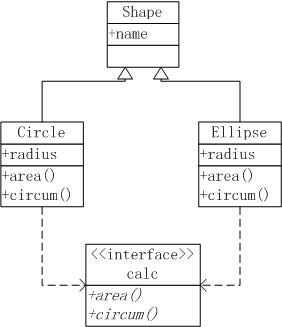``````
# 定义基类Shape
> setClass("Shape",slots=list(name="character"))

> setValidity("Circle",function(object) {
+ })
Class "Circle" [in ".GlobalEnv"]
Slots:
Class:   numeric character
Extends: "Shape"

# 创建两个圆形实例
> c1<-new("Circle",name="c1")
``````

``````
# 计算面积泛型函数接口
> setGeneric("area",function(obj,...) standardGeneric("area"))
 "area"

# 计算面积的函数现实
> setMethod("area","Circle",function(obj,...){
+     print("Area Circle Method")
+ })
 "area"

# 分别计算c1和c2的两个圆形的面积
> area(c1)
 "Area Circle Method"
 3.141593
> area(c2)
 "Area Circle Method"
 78.53982
``````

``````
# 计算周长泛型函数接口
> setGeneric("circum",function(obj,...) standardGeneric("circum"))
 "circum"

# 计算周长的函数现实
> setMethod("circum","Circle",function(obj,...){
+ })

# 分别计算c1和c2的两个圆形的面积
 "circum"
> circum(c1)
 6.283185
> circum(c2)
 31.41593
``````

``````

> setValidity("Ellipse",function(object) {
+     if (length(object@radius) != 2 ) stop("It's not Ellipse.")
+ })
Class "Ellipse" [in ".GlobalEnv"]
Slots:
Class:   numeric character
Extends: "Shape"

# 创建两个椭圆形实例e1,e2
> e1<-new("Ellipse",name="e1")

# 计算椭圆形面积的函数现实
> setMethod("area", "Ellipse",function(obj,...){
+     print("Area Ellipse Method")
+ })
 "area"

# 计算e1,e2两个椭圆形的面积
> area(e1)
 "Area Ellipse Method"
 3.141593
> area(e2)
 "Area Ellipse Method"
 15.70796

# 计算椭圆形周长的函数现实
> setMethod("circum","Ellipse",function(obj,...){
+     cat("Ellipse Circum :\n")
+ })
 "circum"

# 计算e1,e2两个椭圆形的周长
> circum(e1)
Ellipse Circum :
 6.283185
> circum(e2)
Ellipse Circum :
 22.65435
``````

6.2 任务二：重构圆形和椭圆形的设计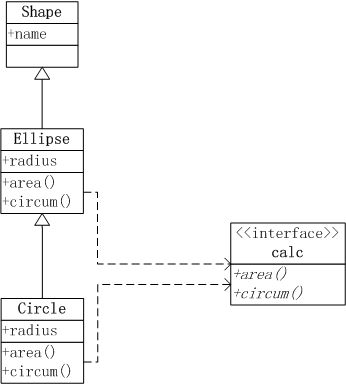``````
# 基类Shape
> setClass("Shape",slots=list(name="character",shape="character"))

# Ellipse继承Shape

# Circle继承Ellipse

# 定义area接口
> setGeneric("area",function(obj,...) standardGeneric("area"))
 "area"

# 定义area的Ellipse实现
> setMethod("area","Ellipse",function(obj,...){
+     cat("Ellipse Area :\n")
+ })
 "area"

# 定义area的Circle实现
> setMethod("area","Circle",function(obj,...){
+     cat("Circle Area :\n")
+ })
 "area"

# 定义circum接口
> setGeneric("circum",function(obj,...) standardGeneric("circum"))
 "circum"

# 定义circum的Ellipse实现
> setMethod("circum","Ellipse",function(obj,...){
+     cat("Ellipse Circum :\n")
+ })
 "circum"

# 定义circum的Circle实现
> setMethod("circum","Circle",function(obj,...){
+     cat("Circle Circum :\n")
+ })
 "circum"

# 创建实例

# 计算椭圆形的面积和周长
> area(e1)
Ellipse Area :
 31.41593
> circum(e1)
Ellipse Circum :
 23.92566

# 计算圆形的面积和周长
> area(c1)
Circle Area :
 12.56637
> circum(c1)
Circle Circum :
 12.56637
``````

6.3 任务三：增加矩形的图形处理

• 定义矩形的数据结构，并计算面积和周长
• 定义正方形的数据结构，并计算面积和周长
• 正方形是矩形的特例，定义矩形是正方形的父类，而正方形是矩形的子类。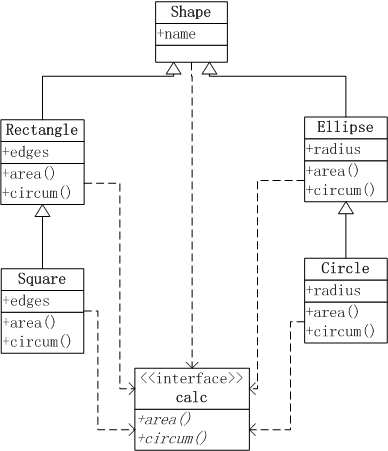``````
# 定义矩形Rectangle，继承Shape
> setClass("Rectangle",contains="Shape",slots=list(edges="numeric"),prototype=list(edges=c(1,1),shape="Rectangle"))

# 定义正方形Square，继承Rectangle
> setClass("Square",contains="Rectangle",slots=list(edges="numeric"),prototype=list(edges=1,shape="Square"))

# 定义area的Rectangle实现
> setMethod("area","Rectangle",function(obj,...){
+     cat("Rectangle Area :\n")
+     prod(obj@edges)
+ })
 "area"

# 定义area的Square实现
> setMethod("area","Square",function(obj,...){
+     cat("Square Area :\n")
+     obj@edges^2
+ })
 "area"

# 定义circum的Rectangle实现
> setMethod("circum","Rectangle",function(obj,...){
+     cat("Rectangle Circum :\n")
+     2*sum(obj@edges)
+ })
 "circum"

# 定义circum的Square实现
> setMethod("circum","Square",function(obj,...){
+     cat("Square Circum :\n")
+     4*obj@edges
+ })
 "circum"

# 创建实例
> r1<-new("Rectangle",name="r1",edges=c(2,5))
> s1<-new("Square",name="s1",edges=2)

# 计算矩形形的面积和周长
> area(r1)
Rectangle Area :
 10
> area(s1)
Square Area :
 4

# 计算正方形的面积和周长
> circum(r1)
Rectangle Circum :
 14
> circum(s1)
Square Circum :
 8
``````

6.4 任务四：在基类Shape中，增加shape属性和getShape方法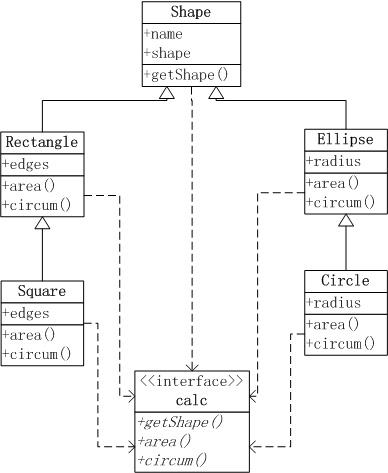``````
# 重新定义基类Shape，增加shape属性
> setClass("Shape",slots=list(name="character",shape="character"))

# 定义getShape接口
> setGeneric("getShape",function(obj,...) standardGeneric("getShape"))
 "getShape"

# 定义getShape实现
> setMethod("getShape","Shape",function(obj,...){
+     cat(obj@shape,"\n")
+ })
 "getShape"
``````

``````
# 实例化一个Square对象，并给shape属性赋值
> s1<-new("Square",name="s1",edges=2, shape="Square")

# 调用基类的getShape()函数
> getShape(r1)
Rectangle
``````

``````
setClass("Rectangle",contains="Shape",slots=list(edges="numeric"),prototype=list(edges=c(1,1),shape="Rectangle"))
setClass("Square",contains="Rectangle",slots=list(edges="numeric"),prototype=list(edges=1,shape="Square"))
``````

``````
# 实例化一个Square对象
> s1<-new("Square",name="s1",edges=2)

# 调用基类的getShape()函数
> getShape(r1)
Rectangle
``````

``````
setClass("Shape",slots=list(name="character",shape="character"))
setClass("Rectangle",contains="Shape",slots=list(edges="numeric"),prototype=list(edges=c(1,1),shape="Rectangle"))
setClass("Square",contains="Rectangle",slots=list(edges="numeric"),prototype=list(edges=1,shape="Square"))

setGeneric("getShape",function(obj,...) standardGeneric("getShape"))
setMethod("getShape","Shape",function(obj,...){
cat(obj@shape,"\n")
})

setGeneric("area",function(obj,...) standardGeneric("area"))
setMethod("area","Ellipse",function(obj,...){
cat("Ellipse Area :\n")
})
setMethod("area","Circle",function(obj,...){
cat("Circle Area :\n")
})
setMethod("area","Rectangle",function(obj,...){
cat("Rectangle Area :\n")
prod(obj@edges)
})
setMethod("area","Square",function(obj,...){
cat("Square Area :\n")
obj@edges^2
})

setGeneric("circum",function(obj,...) standardGeneric("circum"))
setMethod("circum","Ellipse",function(obj,...){
cat("Ellipse Circum :\n")
})
setMethod("circum","Circle",function(obj,...){
cat("Circle Circum :\n")
})
setMethod("circum","Rectangle",function(obj,...){
cat("Rectangle Circum :\n")
2*sum(obj@edges)
})
setMethod("circum","Square",function(obj,...){
cat("Square Circum :\n")
4*obj@edges
})

r1<-new("Rectangle",name="r1",edges=c(2,5))
s1<-new("Square",name="s1",edges=2)

area(e1)
area(c1)
circum(e1)
circum(c1)

area(r1)
area(s1)
circum(r1)
circum(s1)
``````

http://blog.fens.me/r-class-s4/## 撬动R内核的高级工具包pryr

R的极客理想系列文章，涵盖了R的思想，使用，工具，创新等的一系列要点，以我个人的学习和体验去诠释R的强大。

R语言作为统计学一门语言，一直在小众领域闪耀着光芒。直到大数据的爆发，R语言变成了一门炙手可热的数据分析的利器。随着越来越多的工程背景的人的加入，R语言的社区在迅速扩大成长。现在已不仅仅是统计领域，教育，银行，电商，互联网….都在使用R语言。

• 张丹(Conan), 程序员Java,R,PHP,Javascript
• weibo：@Conan_Z
• blog: http://blog.fens.me
• email: bsspirit@gmail.com

http://blog.fens.me/r-pryr/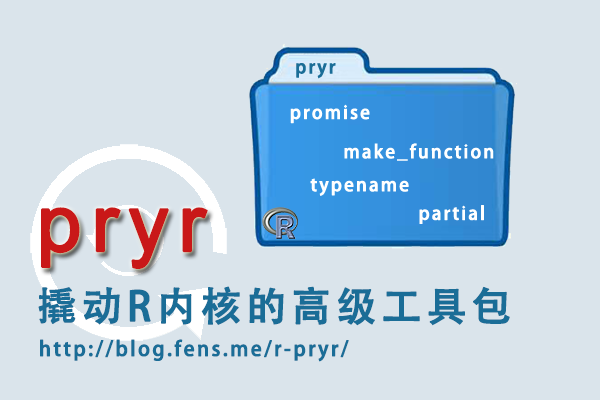1. pryr介绍
2. pryr安装
3. pryr使用

## 1 pryr介绍

pryr包是一个深层的了解R语言运行机制的工具，可以帮助我们更加贴近R语言的核心。为了能开发出更高级R语言应用，需要我们更深入地懂R。

pryr的API介绍

• promise对象：uneval(), is_promise()
• 查询环境变量： where(), rls(), parenv()
• 查看闭包函数变量： unenclose()
• 函数调用关系：call_tree()
• 跟踪对象是否被修改track_copy()

• 判断属于哪种类型对象： otype()
• 判断属于哪种类型函数： ftype()

• 通过参数创建函数：make_function(), f()
• 变量表达式替换：substitute_q(), subs()
• 批量修改对象： modify_lang()
• 快速创建list对象：dots(), named_dots()
• 建匿名函数调用：partial()
• 找符合条件函数：find_funs()

• 创建延迟或直接绑定：%<d-%, %<a-%
• 创建常量绑定：%<c-%
• 重新绑定：rebind, <<-

## 2 pryr安装

• Linux Ubuntu 12.04.2 LTS 64bit
• R 3.0.1 x86_64-pc-linux-gnu (64-bit)

pryr安装

``````
~ R

# 安装devtools
# install.packages("devtools")

> library(devtools)
> install_github("pryr")
``````

## 3 pryr使用

• 3.1 创建匿名函数f()
• 3.2 通过参数创建函数make_function()
• 3.3 创建匿名函数调用partial()
• 3.4 变量表达式替换substitute_q(), subs()
• 3.5 面向对象类型判断otype(),ftype()
• 3.6 查看对象底层的C语言类型 address(), refs(), typename()
• 3.7 查看对象是否被修改track_copy()
• 3.8 查看闭包函数变量 unenclose()
• 3.9 批量修改对象 modify_lang()
• 3.10 快速创建list对象 dots(), named_dots()
• 3.11 查找符合条件函数 fun_calls()
• 3.12 查询环境变量 where(), rls(), parenv()
• 3.13 打印函数调用关系 call_tree(), ast()
• 3.14 promise对象 uneval(), is_promise()
• 3.15 数据绑定%<a-%, %<c-%，%<d-%, rebind,<<-

3.1 创建匿名函数f()

``````
# 创建一个匿名函数
> f(x + y)
function (x, y)
x + y

# 创建一个匿名函数，并赋值计算
> f(x + y)(1, 10)
 11

# 创建一个匿名函数，指定参数和默认值
> f(x, y = 2, x + y)
function (x, y = 2)
x + y

# 创建一个匿名函数，并赋值计算
> f(x, y = 2, x + y)(1)
 3

# 创建一个匿名函数，多行运行，并赋值计算
>  f({y <- runif(1); x + y})(3)
 3.7483
``````

3.2 通过参数创建函数make_function()

make_function()函数的3个参数分别是：

• 生成函数的参数部分, list类型
• 生成函数的表达式部分, 语法表达式, call类型
• 生成函数的系统环境部分, environment类型
``````
# 创建标准的函数
> f <- function(x) x + 3
> f
function(x) x + 3

# 运行函数
> f(12)
 15

# 通过参数创建函数
> g <- make_function(alist(x = ), quote(x + 3))
> g
function (x)
x + 3

# 运行函数
> g(12)
 15
``````

3.3 创建匿名函数调用partial()

``````
# 定义一个普通的函数
> compact1 <- function(x) Filter(Negate(is.null), x)
> compact1
function(x) Filter(Negate(is.null), x)

# 通过partial定义的匿名函数
> compact2 <- partial(Filter, Negate(is.null))
> compact2
function (...)
Filter(Negate(is.null), ...)
``````

``````
# 标准函数实现
> f1 <- function(){runif(rpois(1, 5))}
> f1()
 0.09654228 0.93089395 0.85530142 0.33021067 0.16728877 0.79099825
> f1()
 0.6166580 0.2100876 0.3125176

# 通过partial的匿名函数调用
> f2 <- partial(runif, n = rpois(1, 5))
> f2()
 0.25955143 0.12858459 0.04994997 0.11505708 0.10509429
> f2()
 0.9710866 0.1469317
``````

3.4 变量表达式替换 substitute_q(), subs()

``````
# 定义一个表达式调用
>  x <- quote(a + b)
> class(x)
 "call"

# 对x调用参数替换，无效
>  substitute(x, list(a = 1, b = 2))
x

# 对直接变量参数替换
> substitute(a+b, list(a = 1, b = 2))
1 + 2

# 对x调用参数替换
>  substitute_q(x, list(a = 1, b = 2))
1 + 2

> eval(substitute_q(x, list(a = 1, b = 2)))
 3
``````

``````
> a <- 1
> b <- 2

# 对变量表达式替换，无效
> substitute(a + b)
a + b

# 对变量表达式替换
> subs(a + b)
1 + 2

``````

3.5 面向对象类型判断otype(),ftype()

``````
# 基本类型
> otype(1:10)
 "primitive"
> otype(c('a','d'))
 "primitive"
> otype(list(c('a'),data.frame()))
 "primitive"

# S3类型
> otype(data.frame())
 "S3"

# 自定的S3类型
> x <- 1
> attr(x,'class')<-'foo'
> is.object(x)
 TRUE
> otype(x)
 "S3"

# S4类型
> setClass("Person",slots=list(name="character",age="numeric"))
> alice<-new("Person",name="Alice",age=40)
> isS4(alice)
 TRUE
> otype(alice)
 "S4"

# RC类型
> Account<-setRefClass("Account")
> a<-Account\$new()
> class(a)
 "Account"
attr(,"package")
 ".GlobalEnv"

> is.object(a)
 TRUE
> isS4(a)
 TRUE
> otype(a)
 "RC"
``````

``````
# 标准函数
> ftype(`%in%`)
 "function"

# primitive函数
> ftype(sum)
 "primitive" "generic"

# internal函数
> ftype(writeLines)
 "internal"
> ftype(unlist)
 "internal" "generic"

# S3函数
>  ftype(t.data.frame)
 "s3"     "method"
> ftype(t.test)
 "s3"      "generic"

# S4 函数
> setGeneric("union")
 "union"
> setMethod("union",c(x="data.frame",y="data.frame"),function(x, y){unique(rbind (x, y))})
 "union"
> ftype(union)
 "s4"      "generic"

# RC函数
> Account<-setRefClass("Account",fields=list(balance="numeric"),methods=list(
+   withdraw=function(x){balance<<-balance-x},
+   deposit=function(x){balance<<-balance+x}))
> a<-Account\$new(balance=100)
> a\$deposit(100)
> ftype(a\$deposit)
 "rc"     "method"
``````

• typename: 返回C语言类型名
• refs: 返回指针数字

``````
# 定义一个变量x
>  x <- 1:10

# 打印C语言类型名
> typename(x)
 "INTSXP"

# 返回指针
> refs(x)
 1

# 打印内存地址
 "0x365f560"

# 定义一个list对象
>  z <- list(1:10)

# 打印C语言类型名
>  typename(z)
 "VECSXP"

# 延迟赋值
> delayedAssign("a", 1 + 2)

# 打印C语言类型名
> typename(a)
 "PROMSXP"

# 打印a变量
> a
 3
> typename(a)
 "PROMSXP"

# 定义变量b，与a变量对比
> b<-3
> typename(b)
 "REALSXP"
``````

3.7 查看对象是否被修改track_copy()

``````
# 定义一个变量
> a<-1:3
> a
 1 2 3

# 查看变量的内存地址

# 跟踪变量
> track_a <- track_copy(a)

# 检查变更是否被修改，没有修改
> track_a()

# 给变量赋值
> a <- 3L

# 查看变量的内存地址，发现没有变化

# 检查变量是否被修改，没有修改
>  track_a()

# 再次给变量赋值
> a<-3

# 查看变量的内存地址，内存地址改变
 "0x37f8580"

# 检查变量是否被修改，已被修改，变成一份copy
>  track_a()
a copied
``````

3.8 查看闭包函数变量 unenclose()

``````
# 定义一个嵌套函数power
>  power <- function(exp) {
+      function(x) x ^ exp
+  }

# 调用闭包函数
>  square <- power(2)
>  cube <- power(3)

# 查看square函数，exp变量并显示没有赋值后的结果
> square
function(x) x ^ exp
<environment: 0x4055f28>

# 查看square函数，exp变量显示赋值后的结果
> unenclose(square)
function (x)
x^2

# 执行square函数
> square(3)
 9
``````

3.9 批量修改对象 modify_lang()

``````
# 定义list对象及内部数据
> examples <- list(
+        quote(a <- 5),
+        alist(a = 1, c = a),
+        function(a = 1) a * 10,
+        expression(a <- 1, a, f(a), f(a = a))
+      )

# 查看对象数据
> examples
[]
a <- 5

[]
[]\$a
 1

[]\$c
a

[]
function (a = 1)
a * 10

[]
expression(a <- 1, a, f(a), f(a = a))

# 定义转换函数a_to_b，
>  a_to_b <- function(x) {
+        if (is.name(x) && identical(x, quote(a))) return(quote(b))
+        x
+  }

# 批量修改对象，替换examples对象中，所有的变量a变成变量b
> modify_lang(examples, a_to_b)
[]
b <- 5

[]
[]\$a
 1

[]\$c
b

[]
function (a = 1)
b * 10

[]
expression(b <- 1, b, f(b), f(a = b))
``````

3.10 快速创建list对象 dots(), named_dots()

``````
# 初始化一个变量
> y <- 2

# 创建list对象
> dots(x = 1, y, z = )
\$x
 1

[]
y

\$z

# 查看对象类型
> class(dots(x = 1, y, z = ))
 "list"

# 查看对象的内部结果
> str(dots(x = 1, y, z = ))
List of 3
\$ x: num 1
\$  : symbol y
\$ z: symbol
``````

``````
# 创建list对象
> named_dots(x = 1, y, z =)
\$x
 1

\$y
y

\$z

# 查看对象类型
> class(named_dots(x = 1, y, z =))
 "list"

# 查看对象的内部结果
> str(named_dots(x = 1, y, z =))
List of 3
\$ x: num 1
\$ y: symbol y
\$ z: symbol
``````

3.11 查找符合条件函数 fun_calls()

``````
> find_funs("package:base", fun_calls, "match.fun", fixed = TRUE)
Using environment package:base
 "apply"  "eapply" "Find"   "lapply" "Map"    "mapply" "Negate" "outer"
 "Reduce" "sapply" "sweep"  "tapply" "vapply"

# 查看Map函数，检查是否包括match.fun字符串
> Map
function (f, ...)
{
f <- match.fun(f)
mapply(FUN = f, ..., SIMPLIFY = FALSE)
}
<bytecode: 0x21688e0>
<environment: namespace:base>
``````

``````
> find_funs("package:stats", fun_args, "^FUN\$")
Using environment package:stats
 "ave"                  "dendrapply"

# 查看ave函数源代码，检查参数名是否有FUN字符串
> ave
function (x, ..., FUN = mean)
{
if (missing(...))
x[] <- FUN(x)
else {
g <- interaction(...)
split(x, g) <- lapply(split(x, g), FUN)
}
x
}
<bytecode: 0x2acba70>
<environment: namespace:stats>
``````

3.12 查询环境变量 where(), rls(), parenv()

``````
# 定义一个变量x
> x <- 1
> where("x")
<environment: R_GlobalEnv>

# 查询t.test函数的位置
> where("t.test")
<environment: package:stats>
attr(,"name")
 "package:stats"
attr(,"path")
 "/usr/lib/R/library/stats"

> t.test
function (x, ...)
UseMethod("t.test")
<bytecode: 0x1ae9bc8>
<environment: namespace:stats>

# 查询mean函数的位置
> where("mean")
<environment: base>

# 查询where函数的位置
> where("where")
<environment: package:pryr>
attr(,"name")
 "package:pryr"
attr(,"path")
 "/home/conan/R/x86_64-pc-linux-gnu-library/3.0/pryr"
``````

``````
# 打印当前环境的变量
> ls()
 "a"                "Account"          "alice"            "a_to_b"
 "b"                "compact1"         "compact2"         "examples"
 "f"                "f1"               "f2"               "g"
 "myGeneric"        "my_long_variable" "plot2"            "union"
 "x"                "y"

# 打印所有环境的变量
> rls()
[]
 "a"                          "Account"
 "alice"                      "a_to_b"
 "b"                          ".__C__Account"
 "compact1"                   "compact2"
 ".__C__Person"               "examples"
 "f"                          "f1"
 "f2"                         "g"
 ".__global__"                "myGeneric"
 "my_long_variable"           "plot2"
 ".Random.seed"               ".requireCachedGenerics"
 ".__T__myGeneric:.GlobalEnv" ".__T__union:base"
 "union"                      "x"
 "y"
``````

``````
# 定义一个3层嵌套函数
> adder <- function(x) function(y) function(z) x + y + z

# 调用第一层函数

# 查看函数
function(y) function(z) x + y + z
<environment: 0x323c000>

# 调用第二层函数
function(z) x + y + z
<environment: 0x3203558>

# 查内层函数的上一级环境
<environment: 0x323c000>
<environment: R_GlobalEnv>
``````

3.13 找印调用关系 call_tree(), ast()

``````
# 嵌套函数语句调用
>  call_tree(quote(f(x, 1, g(), h(i()))))
\- ()
\- `f
\- `x
\-  1
\- ()
\- `g
\- ()
\- `h
\- ()
\- `i

# 条件语句调用
> call_tree(quote(if (TRUE) 3 else 4))
\- ()
\- `if
\-  TRUE
\-  3
\-  4

# 表达式语句调用
> call_tree(expression(1, 2, 3))
\-  1
\-  2
\-  3
``````

``````
# 嵌套表达式语句
> ast(f(x, 1, g(), h(i())))
\- ()
\- `f
\- `x
\-  1
\- ()
\- `g
\- ()
\- `h
\- ()
\- `i

# 条件语句
> ast(if (TRUE) 3 else 4)
\- ()
\- `if
\-  TRUE
\-  3
\-  4

# 函数定义
> ast(function(a = 1, b = 2) {a + b})
\- ()
\- `function
\- []
\ a = 1
\ b = 2
\- ()
\- `{
\- ()
\- `+
\- `a
\- `b
\-

# 函数调用
> ast(f()()())
\- ()
\- ()
\- ()
\- `f
``````

3.14 promise对象 uneval(), is_promise()

promise对象：是R语言中延迟加载机制的一部分，包含三个部分：值，表达式和环境。当函数被调用时参数进行匹配，然后每个形式参数会绑定到一个promise上。表达式有形式参数和存储在promise里的函数的指针。

``````
# 定义变量并赋值
> x <- 10

# 检查是否 promise模式
> is_promise(x)
 FALSE

# 匿名函数调用，检查是否 promise模式
> (function(x) is_promise(x))(x = 10)
 TRUE
``````

``````
# 定义一个函数
> f <- function(x) {
+     uneval(x)
+ }

# 打印函数调用
> f(a + b)
a + b

> class(f(a+b))
 "call"

# 打印函数调用
> f(1 + 4)
1 + 4

# 延迟赋值
> delayedAssign("x", 1 + 4)

# 不执行函数调用，只打印函数调用
> uneval(x)
1 + 4

# 执行函数调用，并赋值
> x
 5

# 延迟赋值又一例
> delayedAssign("x", {
+     for(i in 1:3)
+         cat("yippee!\n")
+     10
+ })

# 执行函数调用，并赋值
> x
yippee!
yippee!
yippee!
 10
``````

3.15 数据绑定%<a-%, %<c-%，%<d-%, rebind,<<-

``````
> x %<a-% runif(1)
> x
 0.06793592
> x
 0.8217227
``````

``````
> y %<c-% 4 + 2
 6
> y
 4
``````

``````
> z %<d-% (a + b)
> a <- 10
> b <- 100
> z
 110
``````

``````
# 对已知变量a重新赋值
> a <- 1
> rebind("a", 2)

# 对未知变量cc重新赋值，出错
> rebind("ccc", 2)
Error: Can't find ccc

# 用 <<- 对已知变量a重新赋值
> a<<-2
> a
 2

# 用 <<- 对未知变量cc重新赋值
> rm(ccc)
> ccc